# Swift Algorithm Club: Swift Depth First Search

Learn how to implement the depth-first search algorithm in Swift, in this step by step tutorial with downloadable sample code.

The Swift Algorithm Club is an open source project, where we as a community implement popular data structures and algorithms in Swift.

Every month, the Swift Algorithm Club team will feature a data structure or algorithm from the club in a tutorial format. If you want to learn more about algorithms and data structures, follow along with us!

In this tutorial you’ll walk through a popular search and pathfinding algorithm: depth-first search.

This tutorial assumes you have read our Swift Graphs with Adjacency Lists and Swift Stack Data Structure or have the equivalent knowledge.

If you have been following along with our tutorial series, be sure to check out our breadth first search tutorial; another Swift pathfinding algorithm.

## Getting Started

Have ever you seen the movie “Honey, I Shrunk the Kids“?

Imagine yourself being shrunk down to the size of an ant. You fall into an ant nest, and have to find your way out. What strategy are you going to use?One great algorithm for solving maze problems like this is the Depth-first search algorithm, first developed by Charles Pierre Trémaux.

In this algorithm, you explore a branch as far as possible until you reach the end of the branch. At that point, you backtrack (go back a step), and explore the next available branch, until you find a way out.

In this tutorial you will explore how the depth-first search algorithm works in Swift, and how it differs from Breadth-first search.

There are many use cases for depth-first search such as:

1. Topological sorting
2. Detecting a cycle
3. Pathfinding
4. Finding connected components in a sparse graph
5. And many more!

Today you will gain an understanding of depth-first search in Swift through pathfinding.

## Traversing Breadth-First Search vs Depth-First Search

Imagine the graph below represents a maze, where S is the start location, and E is the end of the maze. Below shows a breadth-first search traversal of the maze.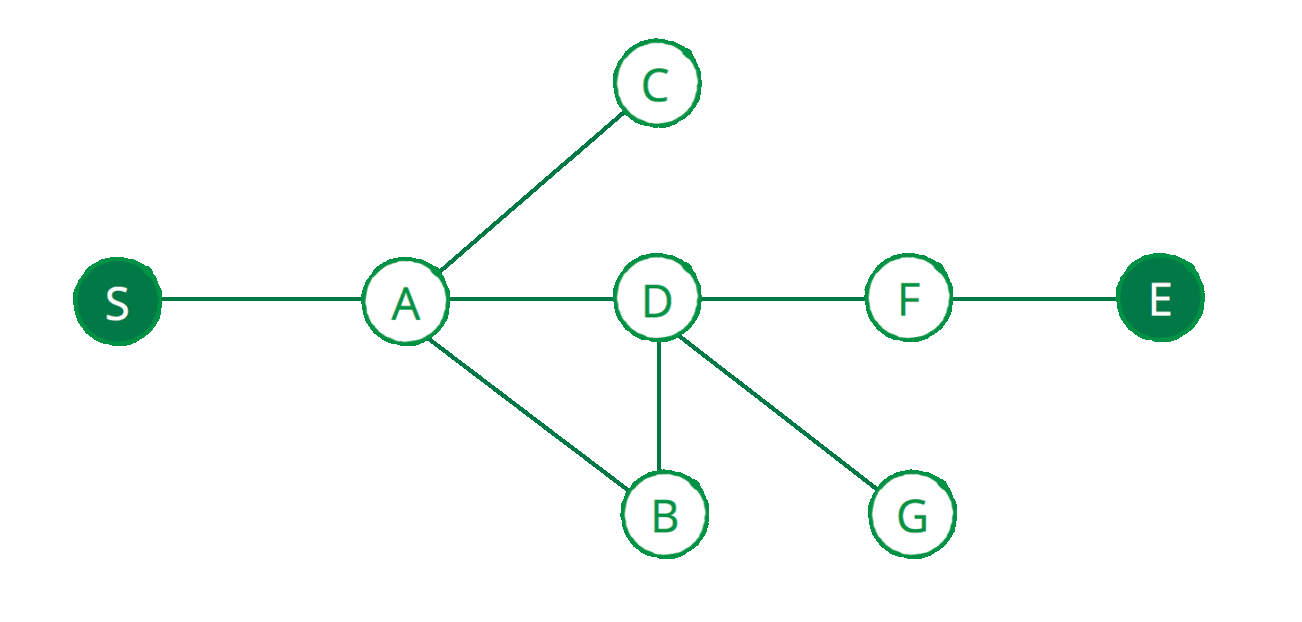On each vertex of the graph, breadth-first search visits every vertex’s immediate neighbors before going to the next. Hence the “breadth” of the traversal.

Next shows how depth-first search traverses the maze.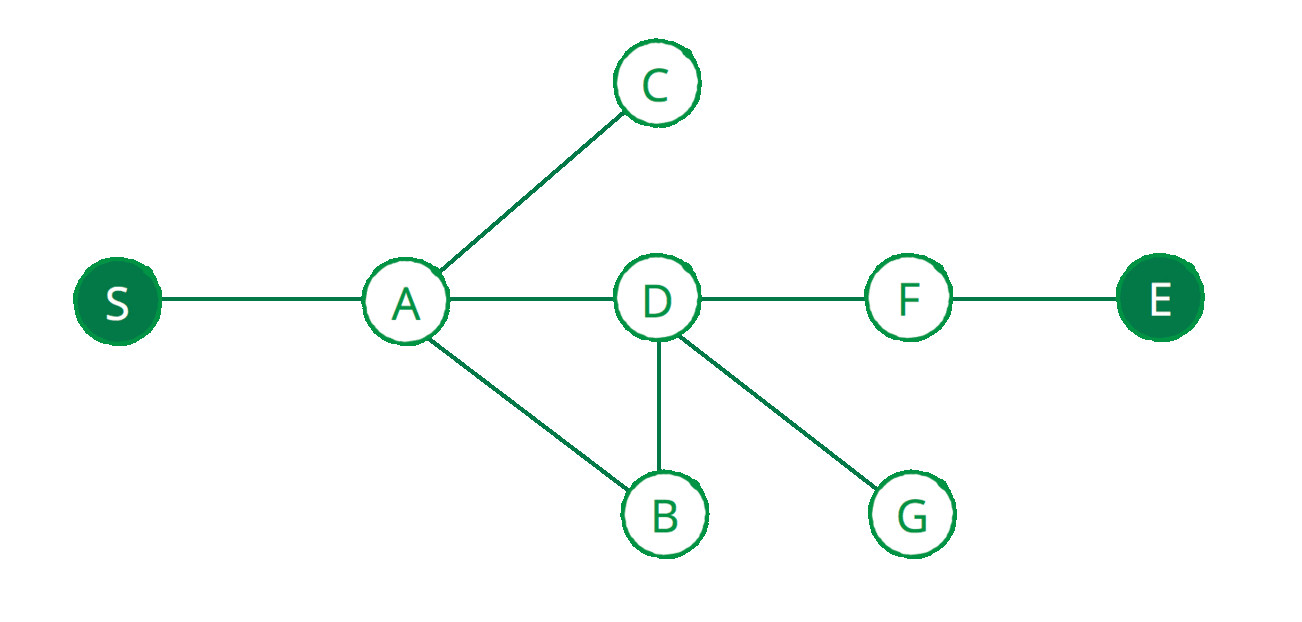Assuming that the algorithm will always traverse the left side first before the right. Depth-first search will start exploring all the vertices on the left-most branch. If the end of the maze is not found, the algorithm will backtrack to the previous vertex, till it finds another path to explore.

For example once node D reaches node G, node G has no other neighbor, hence the backtrack to node D’s next available neighbor node F. Hence the “depth” of the traversal.

## Implementing Depth-First Search

Let’s see what the depth-first search algorithm looks like in Swift.

Start by downloading the starter playground for this tutorial, which has the data structures for a Swift adjacency list and Swift stack included in the Sources group.

Included in the playgrounds is a sample graph used to test the implementation. If you don’t see the diagram, go to Editor > Show Raw Markup to enable it.

Note: If you’re curious how the Swift adjacency list and stack data structures work, you can see the code with View\Navigators\Show Project Navigator. You can also learn how to build these step by step in our Swift Graphs with Adjacency Lists and Swift Stack tutorials.

## Question to Solve

Given a directed or undirected graph, use depth-first search to find a path between two vertices.

### Example

Given the following graph, find a path from S to E: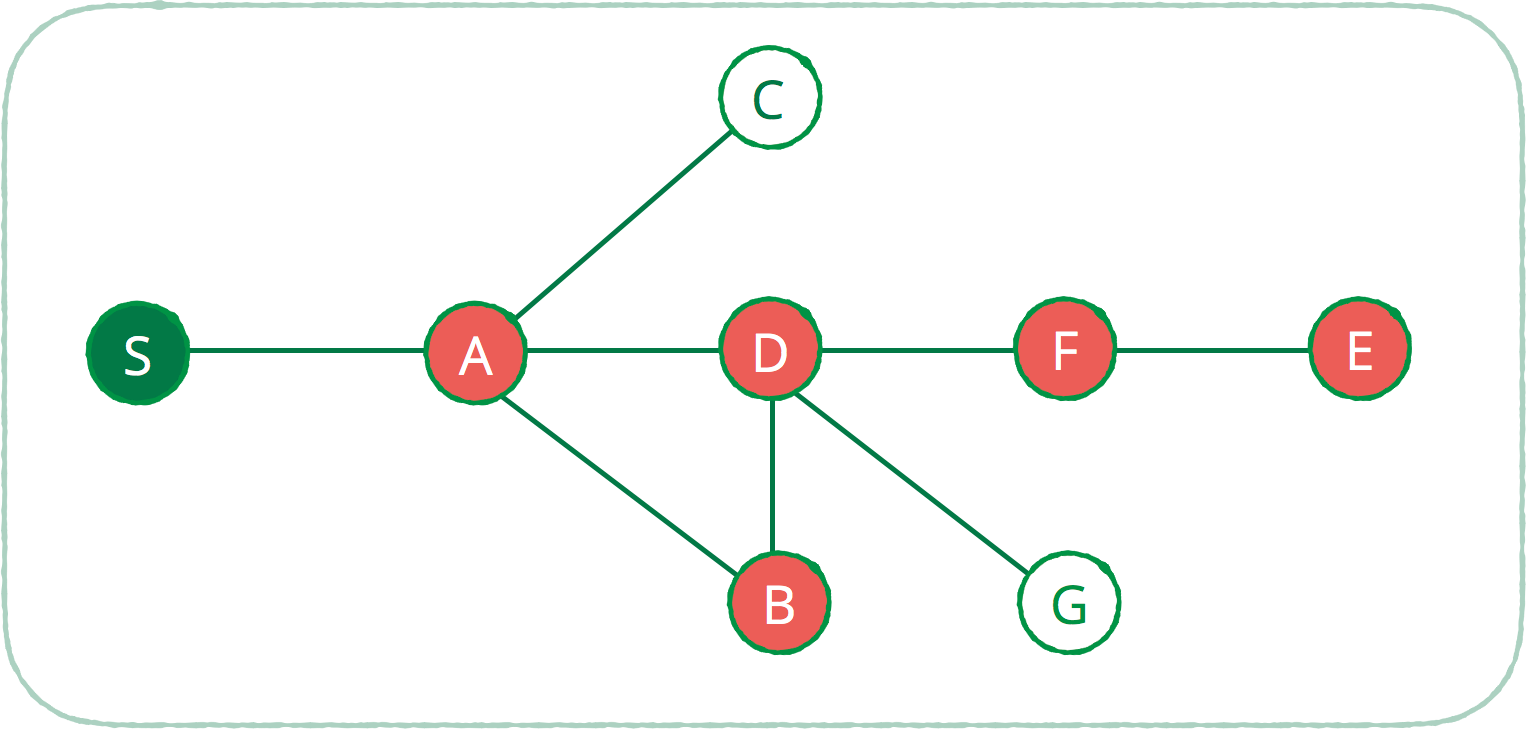The path should be S-A-B-D-F-E

Let’s start the implementation:

Open up the DepthFirstSearch playgrounds, and add the following:

```func depthFirstSearch(from start: Vertex<String>, to end: Vertex<String>, graph: AdjacencyList<String>) -> Stack<Vertex<String>> { // 1
var visited = Set<Vertex<String>>() // 2
var stack = Stack<Vertex<String>>() // 3

// TODO: More code here...

return stack // 4
}
```

Let’s review the snippet above:

1. Defines a function called `depthFirstSearch`, where it takes a start vertex, an end vertex, and the graph you will traverse.
2. Creates a set to store all the vertices that have been visited. This is so we prevent infinite loops.
3. Creates a stack to store a potential path from the start to end vertex.
4. Once the depth-first search completes, the stack will contain the path.

After the TODO, add the following:

```stack.push(start)
visited.insert(start)
```

This initiates the depth-first search by pushing the start node, and marking the start node as visited. Next, add the following code right after:

```outer: while let vertex = stack.peek(), vertex != end { // 1

guard let neighbors = graph.edges(from: vertex), neighbors.count > 0 else { // 2
print("backtrack from \(vertex)")
stack.pop()
continue
}

for edge in neighbors { // 3
if !visited.contains(edge.destination) {
visited.insert(edge.destination)
stack.push(edge.destination)
print(stack.description)
continue outer
}
}

print("backtrack from \(vertex)") // 4
stack.pop()
}
```

Let’s review section by section:

### 1. The outer loop.

```outer: while let vertex = stack.peek(), vertex != end { }
```

The goal is to find a path from the start vertex to the end vertex. So while the stack is not empty, the path has not been found. So begin peeking into the stack’s top vertex, and as long as the vertex is not at the end, check the following:

### 2. Any neighbors?

```guard let neighbors = graph.edges(from: vertex), neighbors.count > 0 else {
print("backtrack from \(vertex)")
stack.pop()
continue
}
```

Check to see if the current vertex has any neighbors. If there are none, that means you reached a dead-end. So backtrack by popping the current vertex off the stack.

### 3. Yes, I have neighbors!

If the vertex has any edges, you are going to loop through each edge.

```for edge in neighbors {
if !visited.contains(edge.destination) { // 1
visited.insert(edge.destination) // 2
stack.push(edge.destination) // 3
print(stack.description) // 4
continue outer // 5
}
}
```

Checking the following:

1. As long as the neighboring vertex has not been visited.
2. Mark the vertex as visited.
3. Push the vertex on the stack. This means that this vertex is a potential candidate for the final path.
4. Print the current path.
5. Continue to the `outer` while loop to branch off the vertex you just pushed. Restarting the whole process from before.

This is depth-first search in action, continuing from the left-most branch.

### 4. All edges visited.

```print("backtrack from \(vertex)")
stack.pop()
```

So what happens if all the edges have been visited? If all the edges from the current vertex has been visited, that means we reached a dead-end. So backtrack and pop it off the stack.

Once the top of the stack contains the end vertex, you have found a path from start to finish!

Finally let’s test out the algorithm. Add the following line at the end of the playground:

```print(depthFirstSearch(from: s, to: e, graph: adjacencyList))
```

This is all you need to implement depth-first search! You should see the following output at the bottom of the playground, displaying the path to the exit:

```E
F
D
B
A
S
```

You think you have depth-first search algorithm nailed down?

## Quiz

From vertex S to vertex C using depth-first search. How many backtracked vertices were there?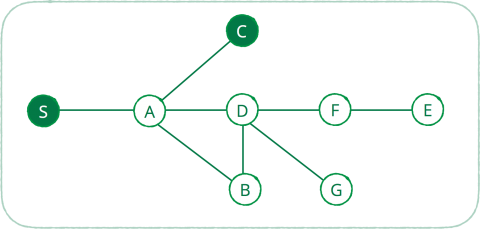[spoiler title=”Solution”]
5 vertices backtracked

backtrack from G
backtrack from E
backtrack from F
backtrack from D
backtrack from B

[/spoiler]

## Where To Go From Here?

Here is the final playground with all the code for the depth-first search algorithm in Swift.

There’s also a recursive version of depth-first search in the Swift Algorithm Club’s repository. So be sure to check that out!

I hope you enjoyed this tutorial on creating pathfinding algorithms with depth-first search in Swift! There are many applications with graphs and this is just one of them.

So stay tuned for many more tutorials from the Swift Algorithm club in the future. In the meantime, if you have any questions or comments, please join the discussion below.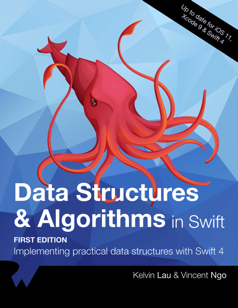If you enjoyed what you learned in this tutorial, why not check out our Data Structures and Algorithms in Swift book, available on our store?

In Data Structures and Algorithms in Swift, you’ll learn how to implement the most popular and useful data structures and when and why you should use one particular datastructure or algorithm over another. This set of basic data structures and algorithms will serve as an excellent foundation for building more complex and special-purpose constructs.

As well, the high-level expressiveness of Swift makes it an ideal choice for learning these core concepts without sacrificing performance.

• You’ll start with the fundamental structures of linked lists, queues and stacks, and see how to implement them in a highly Swift-like way.
• Move on to working with various types of trees, including general purpose trees, binary trees, AVL trees, binary search trees and tries.
• Go beyond bubble and insertion sort with better-performing algorithms, including mergesort, radix sort, heap sort and quicksort.
• Learn how to construct directed, non-directed and weighted graphs to represent many real-world models, and traverse graphs and trees efficiently with breadth-first, depth-first, Dijkstra’s and Prim’s algorithms to solve problems such as finding the shortest path or lowest cost in a network.
• And much, much more!

By the end of this book, you’ll have hands-on experience solving common issues with data structures and algorithms — and you’ll be well on your way to developing your own efficient and useful implementations.